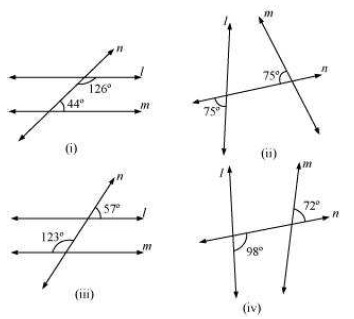# Lines and Angles worksheet for class 7## myCBSEguide App

CBSE, NCERT, JEE Main, NEET-UG, NDA, Exam Papers, Question Bank, NCERT Solutions, Exemplars, Revision Notes, Free Videos, MCQ Tests & more.

## Lines and Angles worksheet for class 7 Important Topics

• Introduction to Lines and Angles
• Related Angles: Complementary and supplementary angles
• Pairs of Lines : Intersecting Line and Transversal
• Check for parallel lines## Some important Facts about Lines and Angles worksheet for class 7

1. We recall that
1. A line-segment has two end points.
2. A ray has only one end point (its vertex); and
3. A line has no end points on either side.
1. When two lines intersect (looking like the letter X) we have two pairs of opposite angles. They are called vertically opposite angles. They are equal in measure.
2. A transversal is a line that intersects two or more lines at distinct points.
3. A transversal gives rise to several types of angles.

## NCERT class 7Mathematics Solved Worksheets

• Chapter 1 – Integers
• Chapter 2 – Fractions and Decimals
• Chapter 3 – Data Handling
• Chapter 4 – Simple Equations
• Chapter 5 – Lines and Angles
• Chapter 6 – Practical Geometry
• Chapter 7 – The Triangle and its Properties
• Chapter 8 – Congruence of triangles
• Chapter 9 – Comparing Quantities
• Chapter 10 – Rational numbers
• Chapter 11 – Perimeter and Area
• Chapter 12 – Algebraic Expressions
• Chapter 13 – Exponents and Powers
• Chapter 14 – Symmetry
• Chapter 15 – Visualizing Solid Shapes

## CHECKING FOR PARALLEL LINES

If two lines are parallel, then you know that a transversal gives rise to pairs of equal corresponding angles, equal alternate interior angles and interior angles on the same side of the transversal being supplementary.

When two lines are given, is there any method to check if they are parallel or not? You need this skill in many life-oriented situations.

A draftsman uses a carpenter’s square and a straight edge (ruler) to draw these segments (Fig 5.30). He claims they are parallel. How? Are you able to see that he has kept the corresponding angles to be equal? (What is the transversal here?)

To download Printable worksheets for class 7 Mathematics and Science; do check myCBSEguide app or website. myCBSEguide provides sample papers with solution, test papers for chapter-wise practice, NCERT solutions, NCERT Exemplar solutions, quick revision notes for ready reference, CBSE guess papers and CBSE important question papers. Sample Paper all are made available through the best app for CBSE students and myCBSEguide website.1. Good